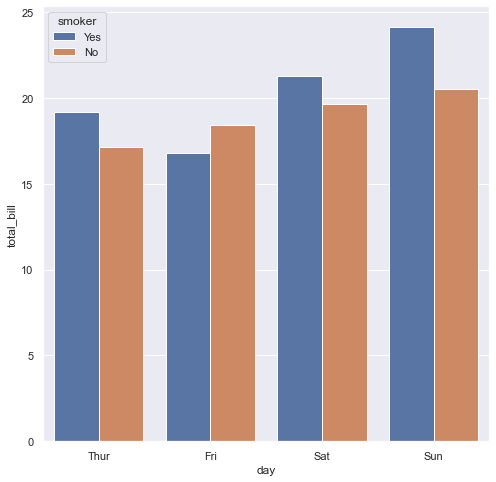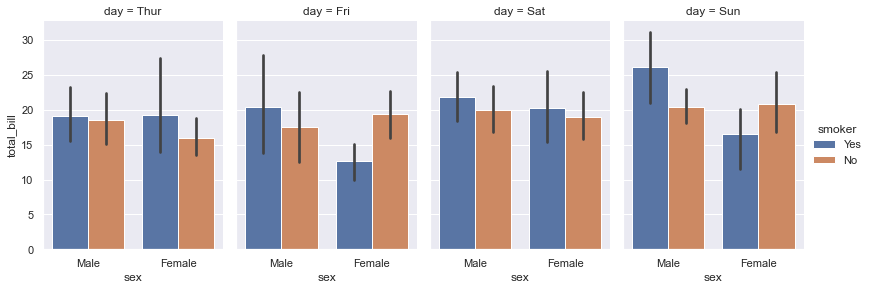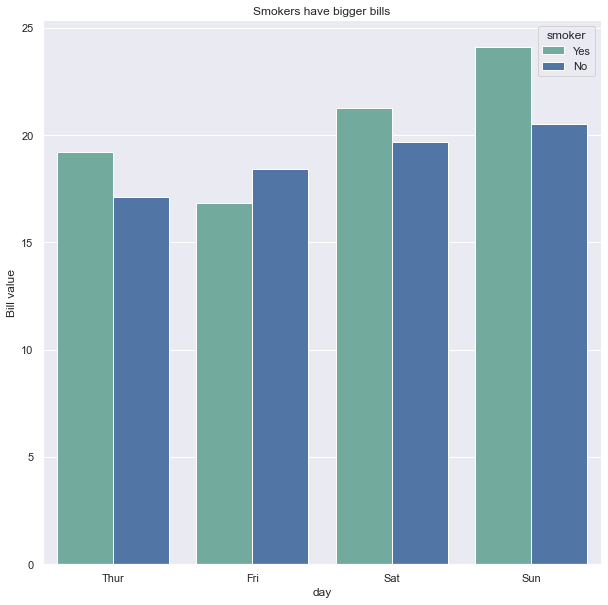# Grouped Barplot using Seaborn

This post explains how to draw a grouped barplot using seaborn. Note that you can check this post to see how to make a basic barplot using seaborn.

## Grouped barplot

Let's consider the total bills paid by a set of people at a restaurant. Those bills are split:

• in groups: the day of the week
• in subgroups: wether the clients were smokers or not

This kind of data allows to build a grouped barplot. Each bar represents the mean bill price for each group and subgroups. The groups are provided the the `x` parameter of the `barplot()` function, the subgroups are passed to the `hue` parameter and will control the color.

``````# import libraries
import seaborn as sns
import matplotlib.pyplot as plt

# set plot style: grey grid in the background:
sns.set(style="darkgrid")

# Set the figure size
plt.figure(figsize=(8, 8))

# grouped barplot
sns.barplot(x="day", y="total_bill", hue="smoker", data=tips, ci=None);``````## More than 2 groups

With more than 2 groups you can use the `catplot()` function to split the plot window. There will be one distinct plot for each of the last subgroup levels.

`````` sns.catplot(x="sex", y="total_bill",
hue="smoker", col="day",
data=tips, kind="bar",
height=4, aspect=.7);``````## Color

You can create an array of colors to use on the chart, pass it to the `color_palette()` function to create a palette with it, and set it as the palette to use thanks to `set_palette()`

``````# Create an array with the colors you want to use
colors = ["#69b3a2", "#4374B3"]
sns.set_palette(sns.color_palette(colors))

# Set the figure size
plt.figure(figsize=(10, 10))

# grouped barplot
ax = sns.barplot(
x="day",
y="total_bill",
hue="smoker",
data=tips,
ci=None
)

# Customize the axes and title
ax.set_title("Smokers have bigger bills")
ax.set_ylabel("Bill value")

# Remove top and right borders
ax.spines['top'].set_visible(False)
ax.spines['right'].set_visible(False)
``````## Contact & Edit

👋 This document is a work by Yan Holtz. Any feedback is highly encouraged. You can fill an issue on Github, drop me a message onTwitter, or send an email pasting `yan.holtz.data` with `gmail.com`.